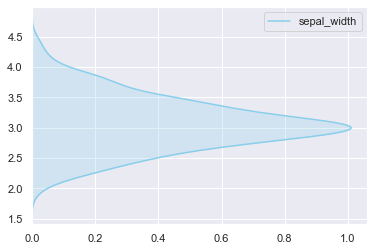It is quite straightforward to build a density plot with a 90 degrees orientation thanks to the 'vertical' parameter.
Note how in the following example, 'vertical' is set to True so that the distribution possible values are located on the y-axis, and their respective count/frequency on the x-axis.

``````# libraries & dataset
import seaborn as sns
import matplotlib.pyplot as plt
# set a grey background (use sns.set_theme() if seaborn version 0.11.0 or above)
sns.set(style="darkgrid")